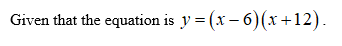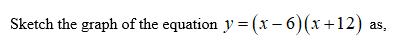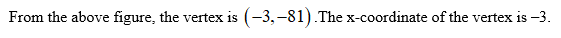# If the equation y=(x-6)(x+12) is graphed in the xy-plane, what is the x-coordinate of the parabola's vertex? A) -6B) -3C) 3D) 6

Question
16 views

If the equation y=(x-6)(x+12) is graphed in the xy-plane, what is the x-coordinate of the parabola's vertex?
A) -6

B) -3

C) 3

D) 6

check_circle

Step 1Step 2Step 3...

### Want to see the full answer?

See Solution

#### Want to see this answer and more?

Solutions are written by subject experts who are available 24/7. Questions are typically answered within 1 hour.*

See Solution
*Response times may vary by subject and question.
Tagged in

### Algebra